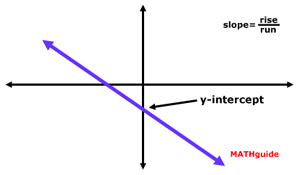Graphing Inequalities: Slope-Intercept Form
View the Lesson | MATHguide homepage Updated April 7th, 2019

Status: Waiting for your answers.Graph the given inequality on your own paper then answer the questions that follow.

 Given: y > - (5/12)x - 6 Find: a) What is the location of the line's y-intercept?Write the location as an ordered pair (x,y).     (, ) b) What is the location of another point on the line?This could be the next point graphed after plotting the y-intercept.     (, ) c) Should the line be solid or dashed?Choose the correct option.     RESPONSE solid dashed d) Should shading occur above or below the line?Choose the correct option.     RESPONSE above below